﻿ 集群型无线传感器网络中基于空间分布的节能方案研究

# 集群型无线传感器网络中基于空间分布的节能方案研究An Effective Energy-Saving Research Based on Spatial Distribution in Clustering WSN

Abstract: Energy consumption caused by energy allocation and data redundancy in cluster-based wireless sensor networks restricts the network scale and energy saving. In this paper, an effective ener-gy-saving scheme based on spatial distribution in clustering WSN (EESC algorithm) is proposed. According to the relative spatial distribution and energy distribution of sensor nodes, head nodes and perceived targets, the sensor nodes of each cluster can be effectively selected. This algorithm can effectively reduce the amount of data sent by the sensor nodes in the cluster to the head node and reduce the risk of data blockage so as to enhance the energy conservation efficiency and im-prove the effectiveness of data fusion. The simulation results show that the method is easy to im-plement, robust and effective, which is superior to classical algorithms.

1. 引言

1) 当测量数据相同的情况下，此时所有传感器节点会向头节点发送相同的数据，这将会导致头节点数据冗余；

2) 网络中发送和接收的冗余数据会造成传感器节点的能量浪费；

3) 发送和接收冗余数据时可能会网络拥堵，且发送和接收无线电信号的能量消耗要远远高出处理其他操作的能量消耗。

1) 研究集群无线传感网的感知策略和分析传感器节点的能量损耗；

2) 提出集群型无线传感器网络中基于空间分布的有效节能方案；

3) 通过仿真模拟该解决方案的节能效果并分析。

2. 感知策略和能量损耗模式

2.1. 感知策略及数据冗余

1) 互补式感知模型

2) 竞争式感知模型

2.2. 传感器节点的能量损耗分析

1) 感知模块

2) 处理模块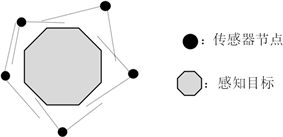Figure 1. Data redundancy source

3) 通信模块

3. 集群型无线传感器网络中基于空间分布的有效节能方案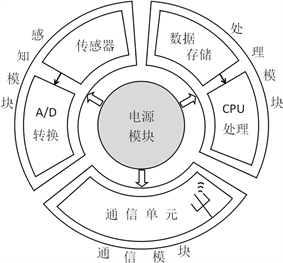Figure 2. Data redundancy source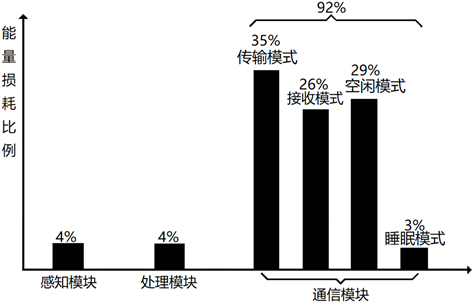Figure 3. Data redundancy source

3.1. 节点的选择标准

${P}_{i}=\alpha \cdot {d}_{i}+\frac{1}{b{p}_{i}}$ (1)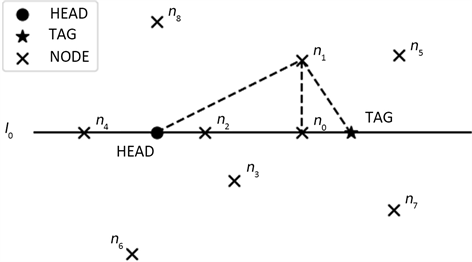Figure 4. The relative position of node, head and tag

3.2. 节点选择率

$R=\frac{\sqrt{{\left(\frac{1}{{N}_{bp}}\underset{i&b{p}_{i}\ne 0}{\sum }{x}_{{n}_{i}}-{x}_{tag}\right)}^{2}+{\left(\frac{1}{{N}_{bp}}\underset{i&b{p}_{i}\ne 0}{\sum }{y}_{{n}_{i}}-{y}_{tag}\right)}^{2}}}{\lambda }×100%$ (2)

 (3)

3.3. 节能算法

1) 输入集群中传感器节点集合 ${\Phi }_{node}$ ；头节点 $Head\left({x}_{head},{y}_{head}\right)$ ；目标节点 $Tag\left({x}_{tag},{y}_{tag}\right)$ ；激活节点集合 ${\Phi }_{act}=\left\{\varnothing \right\}$ ；各个先验参数 $\alpha ,\kappa$ 等；

2) 以 $Tag$ 的连线作为x轴， $Head$ 节点的中点作为y轴建立坐标系，并更新各个节点的坐标；

3) 利用3.1节的节点选择标准方法，根据式(1)计算传感器节点的优先参数P，并按照P从小到大进行排序；

4) 计算集群的集合中心，根据式(2)算出节点选择率R，并由式(3)得出 ${N}_{choose}$

5) 根据3)中排好的顺序从 ${\Phi }_{node}$ 中依次选出节点 ${N}_{choose}$ 个节点组成 ${\Phi }_{act}$

6) 将头节点 $Head$ 加入 ${\Phi }_{act}$

7) ${\Phi }_{act}$ 中的节点激活，感知目标数据并将感知数据发送到 $Head$ 进行数据融合。

4. 仿真分析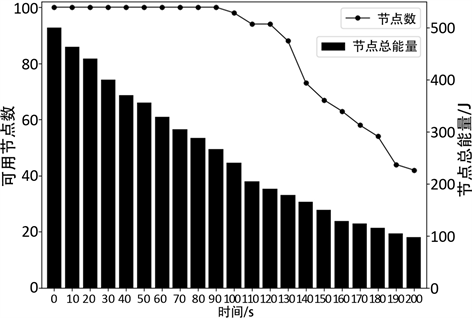Figure 5. The number of available nodes and the total energy of nodes changed over time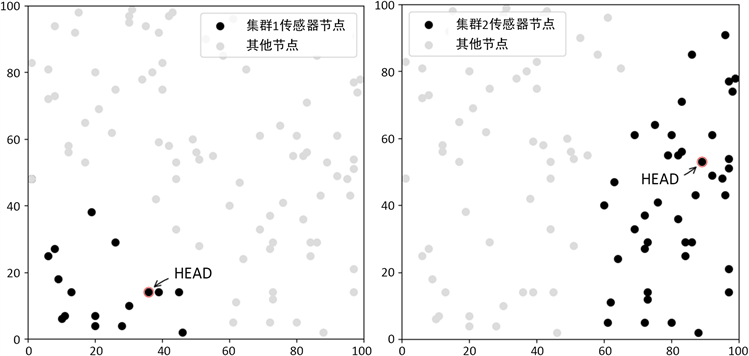(a) (b)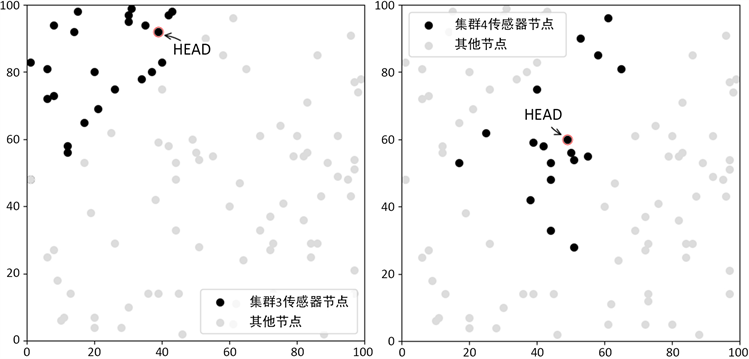(c) (d)

Figure 6. Node location distribution in four clusters

5. 结论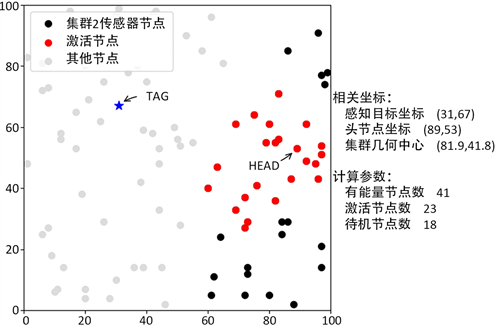Figure 7. Activation node selection (following the EESC)Table 2. Comparison of data transmission between EESC and LEACH

 Jung, W.-S., Lim, K.-W., et al. (2011) Efficient Clustering-Based Data Aggregation Technique for Wireless Sensor Networks. Wireless Networks, 17, 1397-1400.

 Farooq, M.O., Dogar, A.B. and Shah, G. (2010) MR-LEACH: Multi-Hop Routing with Low Energy Adaptive Clustering Hierarchy. Fourth International Conference on Sensor Technologies and Applications (SENSORCOMM), 262-268.

 Izadi, D., Abawajy, J.H., Ghanavati, S. and Herawan, T. (2015) A Data Fusion Method in Wireless Sensor Networks. Sensors, 15, 2964-2979.
https://doi.org/10.3390/s150202964

 Vinan, A.C., Ziviani, A. and Friedman, R. (2009) Decoupling Data Dissemination from Mobile Sink’s Trajectory in Wireless Sensor Network. IEEE Communications Letters, 13, 178-180.
https://doi.org/10.1109/LCOMM.2009.081990

 Leu, J.-S., Chiang, T.-H., Yu, M.-C. and Su, K.-W. (2009) Energy Efficient Clustering Scheme for Prolonging the Lifetime of Wireless Sensor Network with Isolated Nodes. IEEE Communications Letters, 19, 259-262.
https://doi.org/10.1109/LCOMM.2014.2379715

Top